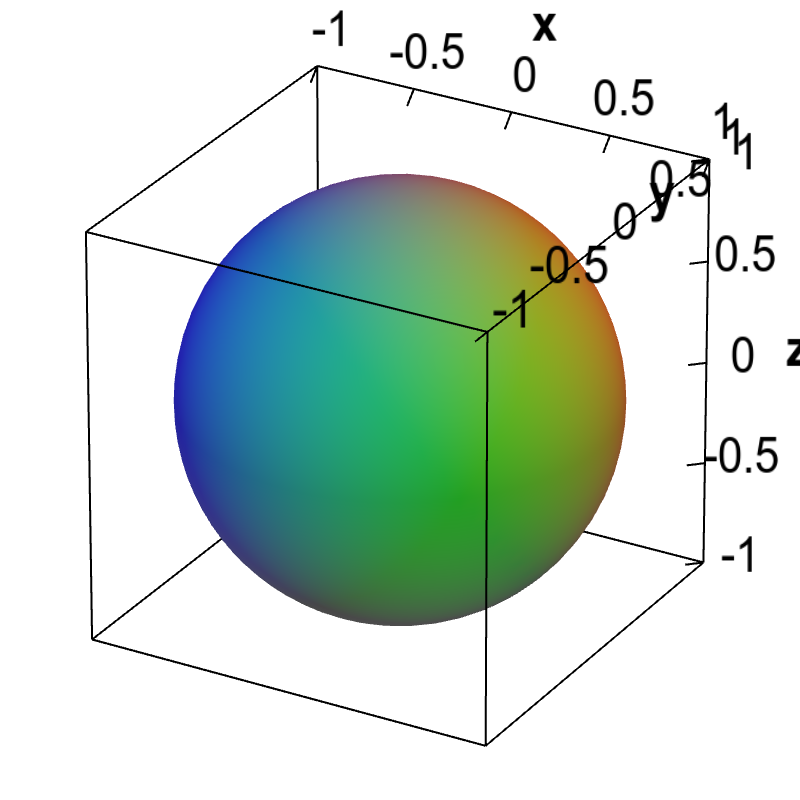# Math Insight

### Surfaces defined implicitly

#### Implicit curves

A graph of a scalar-valued function of a single variable, $y=f(x)$, is a curve. Graph the equation $y=f(x)$ is easy because the value of $y$ is given explicitly for each value of $x$. Even if we aren't told what the function is, we can easily graph an equation such as $y=(x-1)^2$ since it is solved explicitly for $y$.

Sometimes, however, we want to graph an equation such as the equation $x^2+y^2=1$. You might recall that equations like these are called implicit functions, because we can't explicitly solve for $y$ in terms of $x$. If you try to solve this equation for $y$, you get $y = \pm \sqrt{1-x^2}$. The graph of such an equation is still well defined. It is the set of points $(x,y)$ where $x^2+y^2=1$, which is the circle of radius one centered at the origin.

#### Implicit surfaces

We can also graph equations in terms of three variables, say $x$, $y$, and $z$. Sometimes, we can solve the equation explicitly in terms of $z$ and write it as $z=f(x,y)$. In this case, we've reduced the problem to the graph of a scalar-function of two variables, which is a surface.

On the other hand, we cannot solve an equation like $x^2+y^2+z^2=1$ explicitly for $z$. Even so, the equation still implicitly defines a surface. The surface, i.e., the graph of the equation, is the set of points $(x,y,z)$ that satisfy $x^2+y^2+z^2=1$. These points form a sphere of radius one centered at the origin.A spherical implicit surface. The graph of the equation $x^2+y^2+z^2=1$ is the unit sphere centered at the origin.